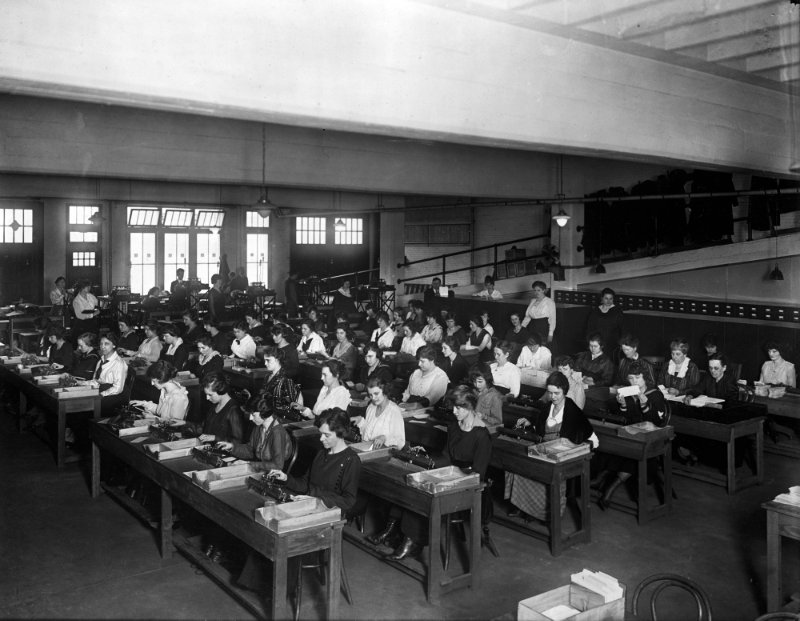September 21st, 2017

## What does Computer means?

A computer is a counter

Normally was a person that did calculations

Sometimes with the help of mechanical devices

During the 2nd World War people invented electronic computers

So, computers are devices handling numbers

## A Computer## “but I don’t use numbers …”

Don’t worry

Using numbers we can represent other things

In my country kids play this game:

They change vowels A, E, I, O, U by the numbers 1, 2, 3, 4, 5

Then they write H2LL4

Using the same idea we can represent any text

## Notice …

… that we have represented sounds by signs for centuries

The key word here is represent

## Numbers can represent other things

There are three things in the Universe

• Matter
• Energy
• Information

Information can be put in digital (numeric) form

• Images
• Audio
• Movies

not yet

• smell
• taste
• tact

## What can a modern computer do?

Computers handle numbers

Numbers represent information

Computers can transform and transfer information

## So, What is a Computer

Computer
(English) counter, calculator
Ordinateur
(French) sorter, gives order to data, handles data
Bilgisayar
(Turkish) Information/Data counter

## Question:

Who invented computers?

## What do you do with a computer?

Do you have a computer at home?

What do you use it for?

## What can a computer do?

• calculate formulas
• solve (some) equations
• store and retrieve huge quantities of data
• find patterns in data
• find data matching a pattern
• transform data in useful ways
• compress data
• move data at low cost without distortion

## Solving an equation

First usage of electronic computers was to solve complex equations

This approach enabled landing on the moon

Let’s find the value $$x$$ that satisfies $24x^3-70x^2+19x+15=0$

## Naming the formula

Let us put a name to the formula. Let’s call it $$f(x)$$. $f(x) = 24x^3-70x^2+19x+15$

We want to find $$x$$ that makes $$f(x)=0.$$ We can write $f(x) = (24x^2-70x+19)x+15$ or even $f(x) = ((24x-70)x+19)x+15$

## Computing f(x) given x

• Take a piece of paper and write $$x$$ in the first line
• Write 24
• Multiply the last two numbers
• Write $$x$$ (from the first line)
• Multiply the last two numbers
• Write $$x$$ (from the first line)
• Multiply the last two numbers
• Compare to 0

## What happened?

We solved a complex mathematical question using a simple set of rules

• write
• multiply
• compare

This decomposition in simple steps is called a program

## Parts of a computer

In this exercise we used

• memory (paper)
• arithmetic/logic units (you: adding, multiplying, deciding)
• input/output (me)

## Programs

Many different questions can be solved with the same rules

It is just a matter of changing the program

First electromechanical computers were like us: A sequence of devices, each one feeding the next

Changing the program required physical change of pieces

## Stored program

### The key step

John Von Neuman realized that the set of steps can be also stored in memory (coded as numbers, obviously)

We only need to include

• a pointer to the current instruction
• a system to decide which arithmetic/logic rule apply

This is called Central Process Unit (CPU)

## Hardware and Software

Since old times physical tools are called hardware

That includes al the physical parts of the computer (what you can kick)

Programs determine the function of the computer, but they are not “physical”.

That is software (what you can only insult)

## Biological analogy

All cell components are hardware

The sequence of the DNA is the software

## What is a computer?

Is a general purpose device that can

• read, process and write numbers
• (and things that can be represented by numbers)
• to and from the memory
• following a program stored also in the memory
• many simple steps

Changing the program changes the purpose of the machine

## In the Next Chapter

### we will see …

• How information is coded in numbers
• How these numbers are stored and organized
• How we interact with computers